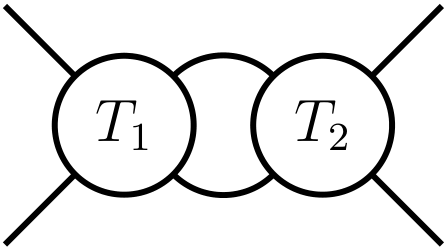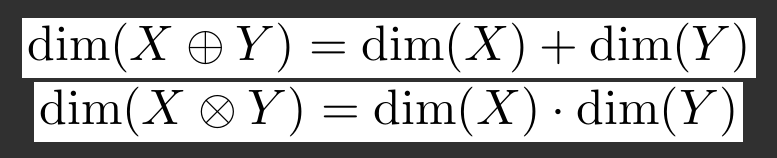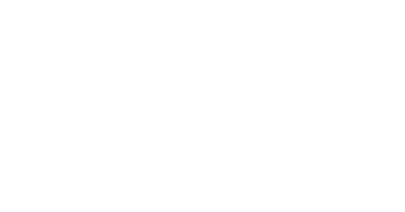# Easy bulk conversion of TikZ diagrams to .pngs

Date written: 2022.05.06

There are benefits to making diagrams in TikZ for use in technical papers. Being able to describe a diagram by a series of drawing commands means vector graphics can be created, controlled, and modified precisely. Simple diagrams can be created very quickly. Once you know the basics, the process of generating simple TiKZ diagrams in LaTeX can be smooth.

For example, I created this diagram from an early paperwith the following TikZ code.

\begin{tikzpicture}[very thick,scale=0.7]
\begin{scope}[xshift=-5cm,rotate around={90:(0,0)}]
\draw (0,1) circle (0.7cm);
\draw (0,-1) circle (0.7cm);
\node (T1) at (0,1) {\large $T_1$};
\node (T2) at (0,-1) {\large $T_2$};
\begin{scope}[yshift=-1cm]
\draw (0,2) ++
(-45:.7)  to [out=-45,in=45,looseness=1]
(45:0.7);
\draw (0,2) ++ (-135:0.7) to [out=-135,in=135,
looseness=1] (135:0.7);
\end{scope}
\draw (0,1) ++ (135:0.7) -- ++ (135:1);
\draw (0,1) ++ (45:0.7) -- ++ (45:1);
\draw (0,-1) ++ (-135:0.7) -- ++ (-135:1);
\draw (0,-1) ++ (-45:0.7) -- ++ (-45:1);
\end{scope}
\end{tikzpicture}


Looking at the code now, there's a couple of things I'd modify to make both the code shorter and easier to read, however the correspondence between the lines of code and the lines which get drawn is still readily apparent.

The fact that the TikZ diagrams are embedded in the final .pdfs means that turning them into other formats in bulk quickly is non-trivial though. Thankfully, there is an easy way to do this using tools which I think are relatively stable.

## Step 1: Convert the diagrams into their own .pdf with the standalone class

The standalone class in LaTeX provides the ability to render arbitary sections into separate .pdfs. For example, the following .tex code

\documentclass[multi={math-to-pdf},border=1pt]{standalone}
\newenvironment{math-to-pdf}{$\displaystyle}{$}
\begin{document}

\begin{math-to-pdf}
\dim (X \oplus Y) = \dim(X) + \dim(Y)
\end{math-to-pdf}

\begin{math-to-pdf}
\dim (X \otimes Y) = \dim(X) \cdot \dim(Y)
\end{math-to-pdf}

\end{document}


produces the following two-page .pdf:By surrounding each equation with a custom environment, and telling the standalone class to use it for rendering, we produced a .pdf where each equation was rendered as its own page. The sizes of these pages are very different to the usual a4 page size in .pdfs, but this does not matter. By surrounding TikZ diagrams with a custom class, we can similarly produce a .pdf where each page is a separate diagram.

## Step 2: Convert pages in a .pdf into separate .png files with ImageMagick

The web is completely and utterly bloated with websites which offer easy conversion of images of various formats to other formats. Some even have the gall to charge money for these services. They are all scams. There is a completely free, open-source command-line tool which can do all of this and more, and which has been around since the early 90s. This tool is ImageMagick

Converting a .png to a .jpeg is completely and utterly trivial with ImageMagick:

The situation for the .pdf is a bit different. While ImageMagick handles conversion between formats for a variety of image types, annoyingly it doesn't actually handle .pdfs by default. Imagemagick needs access to Ghostscript binaries. As such, in addition to downloading and installing ImageMagick I had to download Ghostscript too.

Once this is done, the command

will convert the pages in the .pdf to separate images. The command

displays the image using Imagemagick's own image viewer, which on Windows 11 at the time of writing is IMDisplay. The command

prints out a large amount of metadata about the image; dropping the -verbose argument results in just the essential information (such as the size in pixels and filetype).

For most of the diagrams on my blog, I add in a few more options to the conversion command. Specifically, I increase the resolution of the rendered .pdf, and invert the colors in the diagram. The command

converted the .pdf at the top of this post into the following .pngUnlike the version at the top of this page whose white background is an eyesore, this diagram matches the styling of the text on this page. This command was how I made all the .pngs in my blog on computing the cohomology of the torus from a .pdf of all the diagrams. Since I only had to run the command once, the conversion took no time at all! There was certainly no need to modify the TikZ code to change the color of the rendered diagrams. Imagemagick does this with ease.

Additionally, it is just as easy to turn a number of images into a single pdf where each page is an image: use the corresponding command. There is at least one website which attempts to charge people to do this at scale. Imagemagick does it for free, with as many conversions as you'd like.